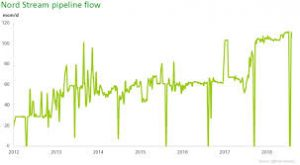## How to Calculate and Solve for Gas Initially in Place of a Reservoir Fluid Flow | The Calculator EncyclopediaThe image above represents gas initially in place.

To compute for gas initially in place, five essential parameters are needed and these parameters are drainage area (A), initial gas FVF (Bgi), initial water saturation (Swi), porosity (φ) and thickness (h).

The formula for calculating gas initially in place:

G = 43560Ah φ (1 – Swi) / Bgi

Where;

G = Gas Initially in Place
A = Drainage Area
Bgi = Initial Gas FVF
Swi = Initial Water Saturation
φ = Porosity
h = Thickness

Let’s solve an example;
Find the gas initially in place with a drainage area of 44, initial gas FVF of 23, initial water saturation of 32, porosity of 18 and exponent of 14.

This implies that;

A = Drainage Area = 44
Bgi = Initial Gas FVF = 23
Swi = Initial Water Saturation = 32
φ = Porosity = 18
h = Thickness = 14

G = 43560Ah φ (1 – Swi) / Bgi
G = 43560 x 44 x 14 x 18 (1 – 32) / 23
G = 43560 x 44 x 14 x 18 (-31) / 23
G = -14972791680 / 23
G = -650990942.6

Therefore, the gas initially in place is -650990942.6